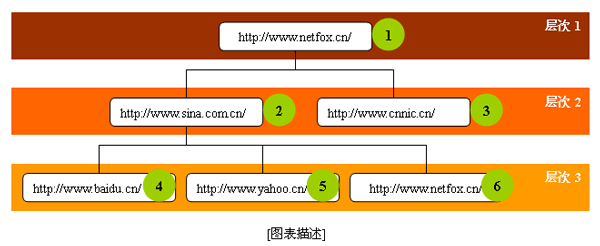# 海纳百川 有容乃大（http://www.brtech.com.cn）

 :: 首页 :: 博问 :: 闪存 :: :: 联系 :: 订阅:: 管理 ::## 蜘蛛程序网站层次及其工作原理描述

 序号 网站 层次 父序号 1 http://www.netfox.cn/ 1 0 2 http://www.sina.com.cn/ 2 1 3 http://www.cnnic.cn/ 2 1 4 http://www.baidu.cn/ 3 2 5 http://www.yahoo.cn/ 3 2

[表格描述]## 蜘蛛程序关键代码

Dim Web(4,10000)        ‘//建立数组

Dim Pointer                  ‘//建立指针，记录当前种子

Dim Id                         ‘//建立序号，记录当前抓区网站的序号

Dim Layer                    ‘//建立层次，记录当前正在运行种子的层次

Dim Running                ‘//建立是否运行的标志，

Private Function NewworkSeed_Set() As Boolean       ‘//用来设置网络种子，为演示方便我们把种子放在数组，

'//当然您也可以根据需要把他们直接放到数据库中

Web(0,0) = 1                                     ‘//序号

Web(1,0) = “http://www.netfox.cn/”           ‘//网站

Web(2,0) = 1                                     ‘//层次

Web(3,0) = 0                                     ‘//父序号，0表示为网络原始种子

Web(4,0) = “奈福网络

‘//当然这里可以设置多个网络原始种子

Web(0,1) = 1

Web(1,1) = “http://www.aspfaq.cn/”

Web(2,1) = 1

Web(3,1) = 0

Web(4,1) = “asp技术站

‘//设置网络种子后，记录种子序号开始后的序号，这里设置了2个种子，所以Id=2开始

Id = 2

End Function

Private Sub Spider_Work()          ‘//蜘蛛工作程序，抓取网站并记录到数组

‘//根据需要可以把他们放到数据库中

Dim A

For Each A In WebBrowser.Document.All

If UCase(A.tagName) = "A" Then

If IsValidWeb(A.href) Then

Id = Id + 1

Web(0, Id) = Id                    ‘//记录当前网站的序号

Web(1, Id) = A.href                     ‘//记录当前网站

Web(2, Id) = Layer               ‘//记录当前网站的层次

If Web(2,Pointer)<> Layer Then Layer = Layer + 1    ‘//当指针层次与当前层次不同的话

‘//则说明层次已经发生了增加

Web(3, Id) = Pointer             ‘//记录当前网站的父序号

Web(4,Id) = A.innerText              ‘//记录当前网站的名称

End If

End If

Next

Pointer = Pointer + 1

WebBrowser.Navigate Web(1, Pointer-1)     ‘//抓取当前种子完毕后，自动跳转到下一个种子

If Running = False Then              ‘//运行为否，退出运行

Exit Sub

End If

End Sub

Private Function Spider_Init() As Boolean    ‘//蜘蛛程序初始化函数

Pointer = 1     ‘//指针设置为1，表示从第一个序号开始运行

Id = 2            ‘//序号设置为2，以后可以读取记录

Layer = 0              ‘//层次设置为0，表示蜘蛛第一次运行

‘//以上指针，序号，层次都可以记录并且方便以后读取

If IsValidWeb(Web(1, Pointer-1)) Then        ‘//判断种子是否正确，如果正确初始化成功，否则失败

Running = True

Spider_Init = True

WebBrowser.Navigate Web(1, Pointer-1)

Else

Running = False

Spider_Init = False

Exit Function

End If

End Function

Private Sub WebBrowser_DocumentComplete(ByVal pDisp As Object, URL As Variant)   ‘//WebBrowser控件

Call Spider_Work()

End Sub

Private Function IsValidWeb(_href) As Boolean           ‘//判断是否是cn域名函数

‘//通过该函数可以实现抓取指定网站或数据

If InStr(_href, "http://www.") > 0 And InStr(_href, ".cn/") > 0 And Len(_href) < 60 Then

IsValidWeb = True

Else

IsValidWeb = False

End If

End Function

Private Sub InitCommand_Click()        ‘//Init初始化 命令控件

If Spider_Init() Then

Msgbox “蜘蛛初始化成功并开始运行了

Else

Msgbox “蜘蛛初始化失败

End If

End Private

Private Sub StopCommand_Click()      ‘//Stop停止 命令控件

Running = False     ‘//停止运行

End Private

Private Sub RunCommand_Click()       ‘//Run运行 命令控件

Running = True      ‘//继续运行

Call Spider_Work()        ‘//蜘蛛运行主程序

End Private

posted on 2006-03-26 06:18 阿昆 阅读(...) 评论(...) 编辑 收藏